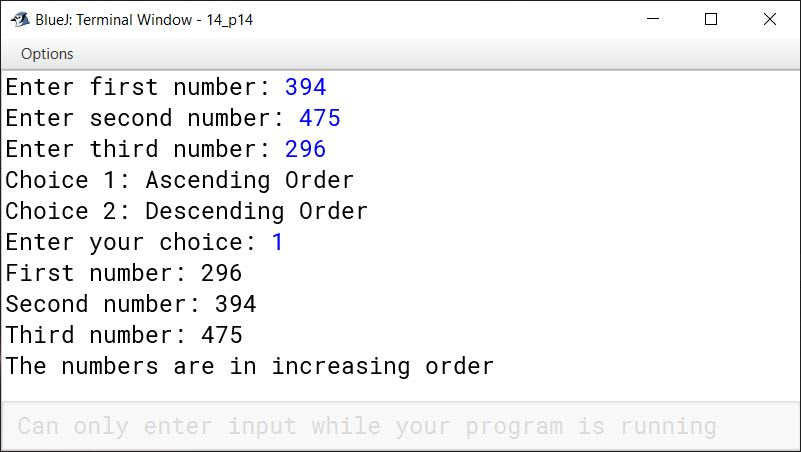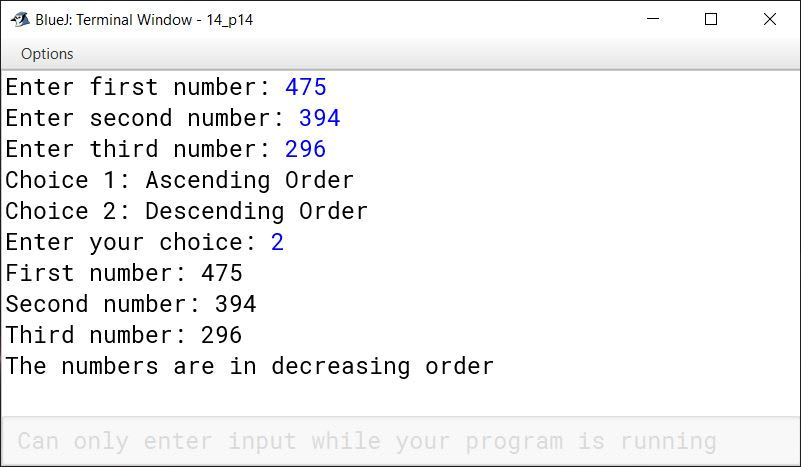# Conditional Statements in Java

## Multiple Choice Questions

#### Question 1

If m, n, p are the three integers, then which of the following holds true, if (m == n) && (n != p)?

1. 'm' and 'n' are equal
2. 'n' and 'p' are equal
3. 'm' and 'p' are equal
4. none

'm' and 'n' are equal

Reason — Assuming that the && operator results in 'true', we can conclude that both the conditions (m == n) and (n != p) are true. Hence, m and n are equal.

#### Question 2

A compound statement can be stated as:

1. p = in.nextInt();
q = in.nextInt();
2. m =+ + a;
n =— b;
3. if(a > b)
{ a++; b--;}
4. none

if(a > b)
{ a++; b--;}

Reason — Two or more statements can be grouped together by enclosing them between opening and closing curly braces. Such a group of statements is called a compound statement.

#### Question 3

If((p>q) && (q>r)) then

1. q is the smallest number
2. q is the greatest number
3. p is the greatest number
4. none

p is the greatest number

Reason — Assuming that the && operator results in 'true', both the conditions (p>q) and (q>r) should be true. Hence, p is the greatest number.

#### Question 4

if(a<b)
c = a;
else
c = b;
It can be written as:

1. c = (b<a) ? a:b;
2. c = (a!=b) ? a:b;
3. c = (a<b) ? b:a;
4. none

none

Reason — Its correct representation using ternary operator is c = (a<b) ? a : b

#### Question 5

If(a < b && a < c)

1. a is the greatest number
2. a is the smallest number
3. b is the greatest number
4. none (where a, b and c are three integer numbers)

a is the smallest number

Reason — Assuming that the && operator results in 'true', both the conditions (a < b) and (a < c) should hold true. Hence a is the smallest of the three numbers.

## State True or False

#### Question 1

if statement is also called as a conditional statement.
True

#### Question 2

A conditional statement is essentially formed by using relational operators.
True

#### Question 3

An if-else construct accomplishes 'fall through'.
False

#### Question 4

In a switch case, when the switch value does not match with any case then the execution transfers to the default case.
True

#### Question 5

The break statement may not be used in a switch statement.
False

## Predict the output

#### Question 1

int m=3,n=5,p=4;
if(m==n && n!=p)
{
System.out.println(m*n);
System.out.println(n%p);
}
if((m!=n) || (n==p))
{
System.out.println(m+n);
System.out.println(m-n);
}

##### Output
8
-2

##### Explanation

The first if condition — if(m==n && n!=p), tests false as m is not equal to n. The second if condition — if((m!=n) || (n==p)) tests true so the statements inside its code block are executed printing 8 and -2 to the console.

#### Question 2

(a) p = 1      (b) p = 3

int a=1,b=2,c=3;
switch(p)
{
case 1: a++;
case 2: ++b;
break;
case 3: c--;
}
System.out.println(a + "," + b + "," +c);

(a) p = 1

##### Output
2,3,3

##### Explanation

When p is 1, case 1 is matched. a++ increments value of a to 2. As there is no break statement, fall through to case 2 happens. ++b increments b to 3. break statement in case 2 transfers program control to println statement and we get the output as 2,3,3.

(b) p = 3

##### Output
1,2,2

##### Explanation

When p is 3, case 3 is matched. c-- decrements c to 2. Program control moves to the println statement and we get the output as 1,2,2.

## Convert the following constructs as directed

#### Question 1

switch case construct into if-else-if :

switch (n)
{
case 1:
s = a + b;
System.out.println("Sum = "+s);
break;
case 2:
d = a - b;
System.out.println("Difference = "+d);
break:
case 3:
p = a * b;
System.out.println("Product = "+p);
break;
default:
System.out.println("Wrong Choice!");
}

if (n == 1) {
s = a + b;
System.out.println("Sum = "+s);
}
else if (n == 2) {
d = a - b;
System.out.println("Difference = "+d);
}
else if (n == 3) {
p = a * b;
System.out.println("Product = "+p);
}
else {
System.out.println("Wrong Choice!");
}

#### Question 2

if-else-if construct into switch case:

if(var == 1)
System.out.println("Distinction");
else if(var == 2)
System.out.println("First Division");
else if(var == 3)
System.out.println("Second Division");
else
System.out.println("invalid"); 

switch (var) {
case 1:
System.out.println("Distinction");
break;
case 2:
System.out.println("First Division");
break;
case 3:
System.out.println("Second Division");
break;
default:
System.out.println("invalid");
}

#### Question 1

What is meant by conditional statement? Explain.

The order in which the statements of a program are executed is known as control flow. By default, the statements of a program are executed from top to bottom in order in which they are written. But most of the times our programs require to alter this top to bottom control flow based on some condition. The statements that help us to alter the control flow of the program are known as conditional statements.

#### Question 2

What is the significance of System.exit(0)?

The function System.exit(0) is used when we want to terminate the execution of the program at any instance. As soon as System.exit(0) function is invoked, it terminates the execution, ignoring the rest of the statements of the program.

Syntax: System.exit(0);

For example, if I am writing a program to find the square root of a number and the user enters a negative number then it is not possible for me to find the square root of a negative number. In such situations, I can use System.exit(0) to terminate the program. The example program to find the square root of a number is given below:

import java.util.Scanner;
public class SquareRootExample {
public static void main(String args[]) {
Scanner in = new Scanner(System.in);
System.out.print("Enter the number: ");
int n = in.nextInt();
if (n < 0) {
System.out.println("Cannot find square root of a negative number");
System.exit(0);
}
double sqrt = Math.sqrt(n);
System.out.println("Square root of " + n + " = " + sqrt);
}
}

#### Question 3

Is it necessary to include default case in a switch statement? Justify.

default case is optional in a switch statement. If no case is matched in the switch block for a given value of control variable, default case is executed implicitly.
Consider the below example:

int number = 4;

switch(number) {

case 0:
System.out.println("Value of number is zero");
break;

case 1:
System.out.println("Value of number is one");
break;

case 2:
System.out.println("Value of number is two");
break;

default:
System.out.println("Value of number is greater than two");
break;

}

Here, value of number is 4 so case 0, case 1 and case 2 are not equal to number. Hence println() of default case will get executed printing "Value of number is greater than two" to the console. If we don't include default case in this example then also the program is syntactically correct but we will not get any output when value of number is anything other than 0, 1 or 2.

#### Question 4

What will happen if 'break' statement is not used in a switch case? Explain.

Use of break statement in a switch case statement is optional. Omitting break statement will lead to fall through where program execution continues into the next case and onwards till end of switch statement is reached.

Consider the below example:

int shape = 2;

switch(number) {

case 0:
System.out.println("Circle");
break;

case 1:
System.out.println("Triangle");

case 2:
System.out.println("Square");

case 3:
System.out.println("Rectangle");

case 4:
System.out.println("Pentagon");
break;

default:
break;

}

Here, we have omitted break statement in cases 1, 2 and 3. When the program executes with the value of control variable as 2, 'Square' will be printed on the screen. Since no break statement is encountered, 'Rectangle' and ' Pentagon' will also be printed. Finally, in case 4 break statement will act as a case terminator and the control will come out of the switch case.

#### Question 5

When does 'Fall through' occur in a switch statement? Explain.

break statement at the end of case is optional. Omitting break leads to program execution continuing into the next case and onwards till a break statement is encountered or end of switch is reached. This is termed as Fall Through in switch case statement.

Consider the below example:

int day = 4;

switch(number) {

case 1:
System.out.println("Monday");

case 2:
System.out.println("Tuesday");

case 3:
System.out.println("Wednesday");

case 4:
System.out.println("Thursday");

case 5:
System.out.println("Friday");

case 6:
System.out.println("Saturday");

case 7:
System.out.println("Sunday");

default:
System.out.println("Invalid choice");
}

Output:

Thursday
Friday
Saturday
Sunday
Invalid choice


#### Explanation

Here, we have omitted break statement in all the cases. When the program executes with the control variable 4, the program prints 'Thursday' and falls through case 5, 6, 7 and default. Thus, 'Friday', 'Saturday', 'Sunday' and 'Invalid choice' gets printed on the output screen.

#### Question 6

What is a compound statement? Give an example.

Two or more statements can be grouped together by enclosing them between opening and closing curly braces. Such a group of statements is called a compound statement. For example,

if (a < b) {

/*
* All statements within this set of braces
* form the compound statement
*/

System.out.println("a is less than b");
a = 10;
b = 20;
System.out.println("The value of a is " + a);
System.out.println("The value of b is " + b);

}

#### Question 7

Explain if-else-if construct with an example.

if-else-if construct is used to test multiple conditions and then take a decision. It provides multiple branching of control.

Below is an example of if-else-if:

if (marks < 35)
System.out.println("Fail");
else if (marks < 60)
else if (marks < 80)
else if (marks < 95)
else
System.out.println("A+ grade");

#### Question 8

Give two differences between the switch statement and the if-else statement.

switchif-else
switch can only test if the expression is equal to any of its case constantsif-else can test for any boolean expression like less than, greater than, equal to, not equal to, etc.
It is a multiple branching flow of control statementIt is a bi-directional flow of control statement

## Solutions to Unsolved Java Programs

#### Question 1

Write a program to input three numbers (positive or negative). If they are unequal then display the greatest number otherwise, display they are equal. The program also displays whether the numbers entered by the user are 'All positive', 'All negative' or 'Mixed numbers'.
Sample Input: 56, -15, 12
Sample Output:
The greatest number is 56
Entered numbers are mixed numbers.

import java.util.Scanner;

public class KboatNumberAnalysis
{
public static void main(String args[]) {

Scanner in = new Scanner(System.in);

System.out.print("Enter first number: ");
int a = in.nextInt();

System.out.print("Enter second number: ");
int b = in.nextInt();

System.out.print("Enter third number: ");
int c = in.nextInt();

int greatestNumber = a;

if ((a == b) && (b == c)) {
System.out.println("Entered numbers are equal.");
}
else {

if (b > greatestNumber) {
greatestNumber = b;
}

if (c > greatestNumber) {
greatestNumber = c;
}

System.out.println("The greatest number is " + greatestNumber);

if ((a >= 0) && (b >= 0) && (c >= 0)) {
System.out.println("Entered numbers are all positive numbers.");
}
else if((a < 0) && (b < 0) && (c < 0)) {
System.out.println("Entered numbers are all negative numbers.");
}
else {
System.out.println("Entered numbers are mixed numbers.");
}
}
}
}
##### Output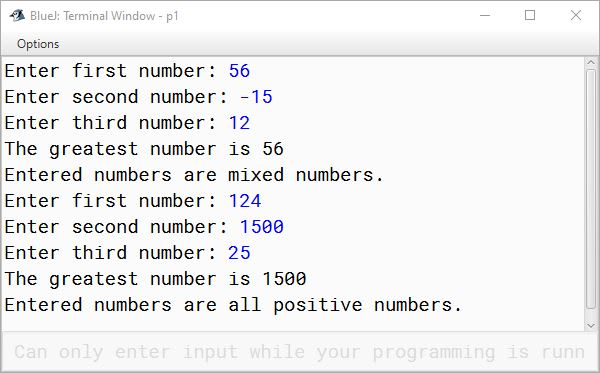#### Question 2

A triangle is said to be an 'Equable Triangle', if the area of the triangle is equal to its perimeter. Write a program to enter three sides of a triangle. Check and print whether the triangle is equable or not.
For example, a right angled triangle with sides 5, 12 and 13 has its area and perimeter both equal to 30.

import java.util.Scanner;

public class KboatEquableTriangle
{
public static void main(String args[]) {

Scanner in = new Scanner(System.in);

System.out.println("Please enter the 3 sides of the triangle.");

System.out.print("Enter the first side: ");
double a = in.nextDouble();

System.out.print("Enter the second side: ");
double b = in.nextDouble();

System.out.print("Enter the third side: ");
double c = in.nextDouble();

double p = a + b + c;
double s = p / 2;
double area = Math.sqrt(s * (s - a) * (s - b) * (s - c));

if (area == p)
System.out.println("Entered triangle is equable.");
else
System.out.println("Entered triangle is not equable.");
}
}
##### Output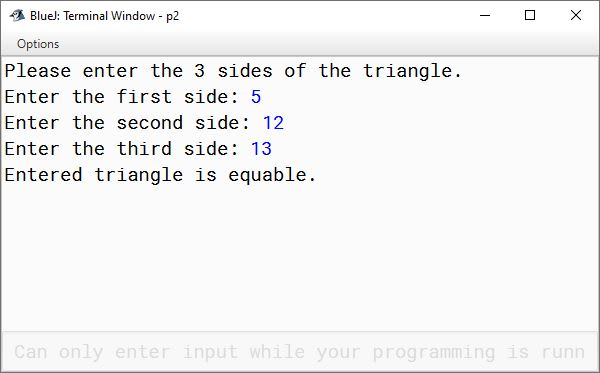#### Question 3

A special two-digit number is such that when the sum of its digits is added to the product of its digits, the result is equal to the original two-digit number.
Example: Consider the number 59.
Sum of digits = 5 + 9 = 14
Product of digits = 5 * 9 = 45
Total of the sum of digits and product of digits = 14 + 45 = 59
Write a program to accept a two-digit number. Add the sum of its digits to the product of its digits. If the value is equal to the number input, display the message "Special 2 - digit number" otherwise, display the message "Not a special two-digit number".

import java.util.Scanner;

public class KboatSpecialNumber
{
public static void main(String args[]) {

Scanner in = new Scanner(System.in);

System.out.print("Enter a 2 digit number: ");
int orgNum = in.nextInt();

if (orgNum < 10 || orgNum > 99) {
System.out.println("Invalid input. Entered number is not a 2 digit number");
System.exit(0);
}

int num = orgNum;

int digit1 = num % 10;
int digit2 = num / 10;
num /= 10;

int digitSum = digit1 + digit2;
int digitProduct = digit1 * digit2;
int grandSum = digitSum + digitProduct;

if (grandSum == orgNum)
System.out.println("Special 2-digit number");
else
System.out.println("Not a special 2-digit number");

}
}
##### Output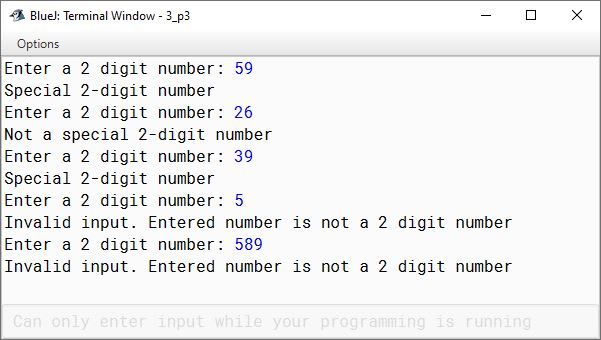#### Question 4

The standard form of quadratic equation is given by: ax2 + bx + c = 0, where d = b2 - 4ac, is known as discriminant that determines the nature of the roots of the equation as:

ConditionNature
if d >= 0Roots are real
if d < 0Roots are imaginary

Write a program to determine the nature and the roots of a quadratic equation, taking a, b, c as input. If d = b2 - 4ac is greater than or equal to zero, then display 'Roots are real', otherwise display 'Roots are imaginary'. The roots are determined by the formula as:
r1 = (-b + √(b2 - 4ac)) / 2a , r2 = (-b - √(b2 - 4ac)) / 2a

import java.util.Scanner;

{
public static void main(String args[]) {

Scanner in = new Scanner(System.in);

System.out.print("Enter a: ");
int a = in.nextInt();

System.out.print("Enter b: ");
int b = in.nextInt();

System.out.print("Enter c: ");
int c = in.nextInt();

double d = Math.pow(b, 2) - (4 * a * c);

if (d >= 0) {
System.out.println("Roots are real.");
double r1 = (-b + Math.sqrt(d)) / (2 * a);
double r2 = (-b - Math.sqrt(d)) / (2 * a);

System.out.println("Roots of the equation are:");
System.out.println("r1=" + r1 + ", r2=" + r2);
}
else {
System.out.println("Roots are imaginary.");
}

}
}
##### Output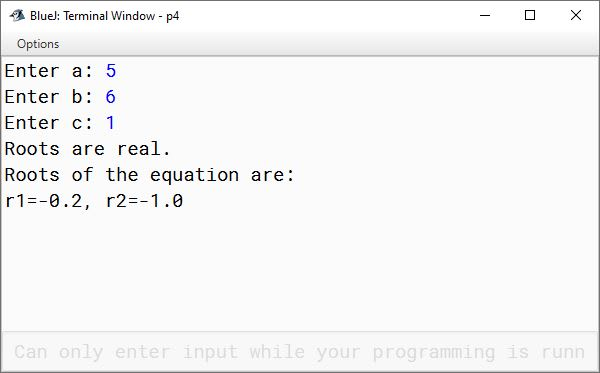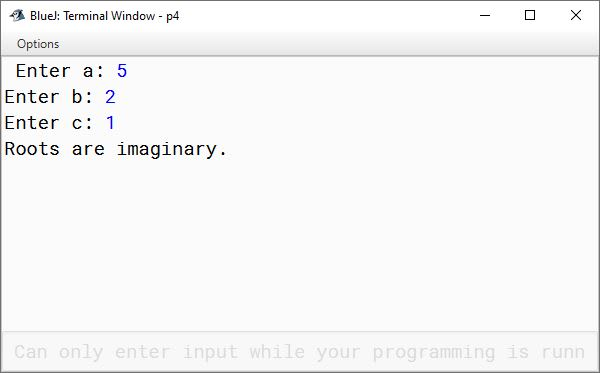#### Question 5

An air-conditioned bus charges fare from the passengers based on the distance travelled as per the tariff given below:

Distance TravelledFare
Up to 10 kmFixed charge ₹80
11 km to 20 km₹6/km
21 km to 30 km₹5/km
31 km and above₹4/km

Design a program to input distance travelled by the passenger. Calculate and display the fare to be paid.

import java.util.Scanner;

public class KboatBusFare
{
public static void main(String args[]) {

Scanner in = new Scanner(System.in);

System.out.print("Enter distance travelled: ");
int dist = in.nextInt();

int fare = 0;

if (dist <= 0)
fare = 0;
else if (dist <= 10)
fare = 80;
else if (dist <= 20)
fare = 80 + (dist - 10) * 6;
else if (dist <= 30)
fare = 80 + 60 + (dist - 20) * 5;
else if (dist > 30)
fare = 80 + 60 + 50 + (dist - 30) * 4;

System.out.println("Fare = " + fare);
}
}
##### Output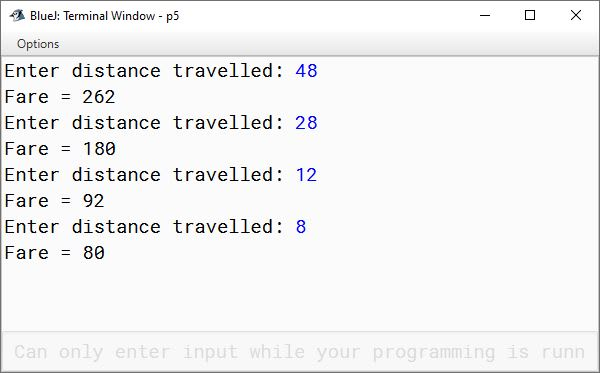#### Question 6

An ICSE school displays a notice on the school notice board regarding admission in Class XI for choosing stream according to marks obtained in English, Maths and Science in Class 10 Council Examination.

Marks obtained in different subjectsStream
Eng, Maths and Science >= 80%Pure Science
Eng and Science >= 80%, Maths >= 60%Bio. Science
Eng, Maths and Science >= 60%Commerce

Print the appropriate stream allotted to a candidate. Write a program to accept marks in English, Maths and Science from the console.

import java.util.Scanner;

{
public static void main(String args[]) {

Scanner in = new Scanner(System.in);

System.out.print("Enter marks in English: ");
int eng = in.nextInt();

System.out.print("Enter marks in Maths: ");
int maths = in.nextInt();

System.out.print("Enter marks in Science: ");
int sci = in.nextInt();

if (eng >= 80 && sci >= 80 && maths >= 80)
System.out.println("Pure Science");
else if (eng >= 80 && sci >= 80 && maths >= 60)
System.out.println("Bio. Science");
else if (eng >= 60 && sci >= 60 && maths >= 60)
System.out.println("Commerce");
else
System.out.println("Cannot allot stream");
}
}
##### Output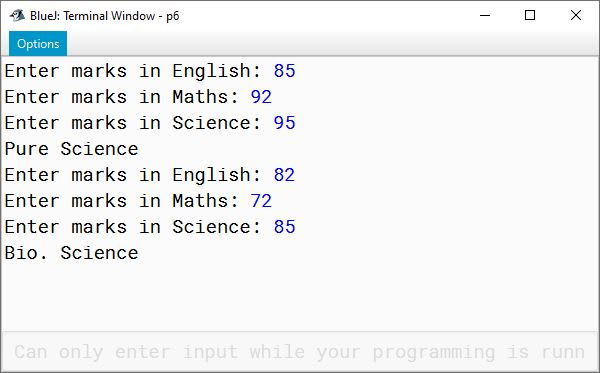#### Question 7

A bank announces new rates for Term Deposit Schemes for their customers and Senior Citizens as given below:

TermRate of Interest (General)Rate of Interest (Senior Citizen)
Up to 1 year7.5%8.0%
Up to 2 years8.5%9.0%
Up to 3 years9.5%10.0%
More than 3 years10.0%11.0%

The 'senior citizen' rates are applicable to the customers whose age is 60 years or more. Write a program to accept the sum (p) in term deposit scheme, age of the customer and the term. The program displays the information in the following format:

Amount DepositedTermAgeInterest earnedAmount Paid
xxxxxxxxxxxxxxx
import java.util.Scanner;

public class KboatBankDeposit
{
public static void main(String args[]) {

Scanner in = new Scanner(System.in);

System.out.print("Enter sum: ");
double sum = in.nextDouble();

System.out.print("Enter age: ");
int age = in.nextInt();

System.out.print("Enter term: ");
double term = in.nextDouble();

double si = 0.0;

if (term <= 1 && age < 60)
si = (sum * 7.5 * term) / 100;
else if (term <= 1 && age >= 60)
si = (sum * 8.0 * term) / 100;
else if (term <= 2 && age < 60)
si = (sum * 8.5 * term) / 100;
else if (term <= 2 && age >= 60)
si = (sum * 9.0 * term) / 100;
else if (term <= 3 && age < 60)
si = (sum * 9.5 * term) / 100;
else if (term <= 3 && age >= 60)
si = (sum * 10.0 * term) / 100;
else if (term > 3 && age < 60)
si = (sum * 10.0 * term) / 100;
else if (term > 3 && age >= 60)
si = (sum * 11.0 * term) / 100;

double amt = sum + si;

System.out.println("Amount Deposited: " +  sum);
System.out.println("Term: " +  term);
System.out.println("Age: " +  age);
System.out.println("Interest Earned: " +  si);
System.out.println("Amount Paid: " +  amt);
}
}
##### Output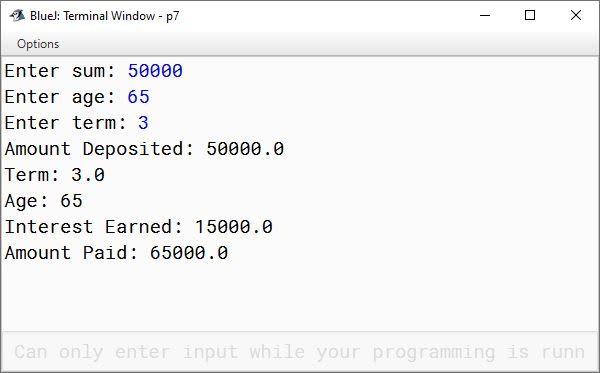#### Question 8

A courier company charges differently for 'Ordinary' booking and 'Express' booking based on the weight of the parcel as per the tariff given below:

Weight of parcelOrdinary bookingExpress booking
Up to 100 gm₹80₹100
101 to 500 gm₹150₹200
501 gm to 1000 gm₹210₹250
More than 1000 gm₹250₹300

Write a program to input weight of a parcel and type of booking (O' for ordinary and 'E' for express). Calculate and print the charges accordingly.

import java.util.Scanner;

public class KboatCourierCompany
{
public static void main(String args[]) {

Scanner in = new Scanner(System.in);

System.out.print("Enter weight of parcel: ");
double wt = in.nextDouble();

System.out.print("Enter type of booking: ");
char type = in.next().charAt(0);

double charge = 0;

if (wt <= 0)
charge = 0;
else if (wt <= 100 && type == 'O')
charge = 80;
else if (wt <= 100 && type == 'E')
charge = 100;
else if (wt <= 500 && type == 'O')
charge = 150;
else if (wt <= 500 && type == 'E')
charge = 200;
else if (wt <= 1000 && type == 'O')
charge = 210;
else if (wt <= 1000 && type == 'E')
charge = 250;
else if (wt > 1000 && type == 'O')
charge = 250;
else if (wt > 1000 && type == 'E')
charge = 300;

System.out.println("Parcel charges = " + charge);
}
}
##### Output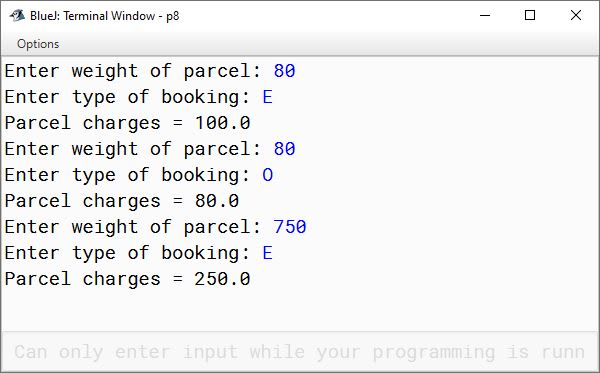#### Question 9

Write a program to input a number and check whether it is a perfect square or not. If the number is not a perfect square then find the least number to be added to input number, so that the resulting number is a perfect square.
Example 1:
Sample Input: 2025
√2025 = 45
Sample Output: It is a perfect square.
Example 2:
Sample Input: 1950
√1950 = 44.1588........
Least number to be added = 452 - 1950 = 2025 - 1950 = 75

import java.util.*;

public class KboatPerfectSquare
{
public static void main(String args[])
{
Scanner in = new Scanner(System.in);
System.out.print("Enter a number: ");
int num = in.nextInt();

if(num < 0) {
System.out.println("Cannot calculate square root of negative number.");
System.exit(0);
}
else
{
double sqRoot = Math.sqrt(num);
double sqRoot1 = Math.floor(sqRoot);
double diff = sqRoot - sqRoot1;
if (diff == 0) {
System.out.println(num + " is a perfect square.");
}
else {
sqRoot1 += 1;
double temp = Math.pow(sqRoot1,2);
diff = temp - num;
System.out.println(num + " is not a perfect square.");
System.out.println("Least number to be added = " + diff);
}
}
}
}
##### Output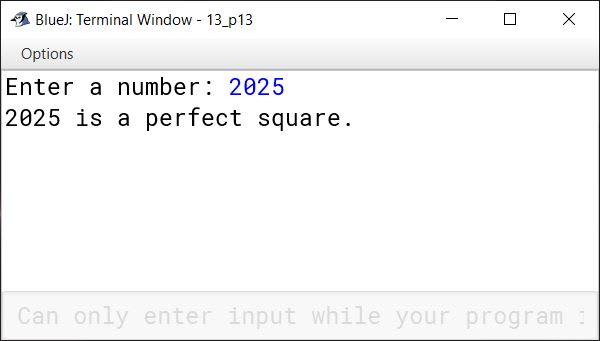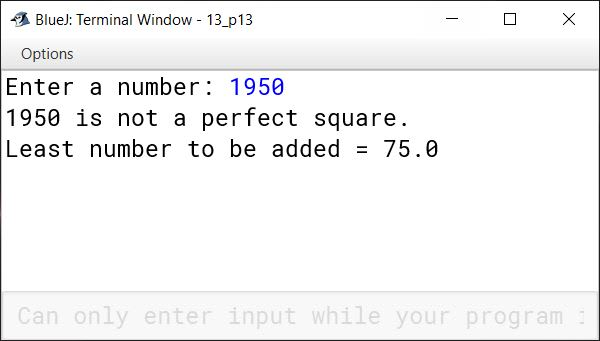#### Question 10

Write a program that accepts three numbers from the user and displays them either in "Increasing Order" or in "Decreasing Order" as per the user's choice.
Choice 1: Ascending order
Choice 2: Descending order

Sample Inputs: 394, 475, 296
Choice 2
Sample Output
First number : 475
Second number: 394
Third number : 296
The numbers are in decreasing order.

import java.util.*;

public class KboatSortNumbers
{
public static void main(String args[])
{
Scanner in = new Scanner(System.in);
System.out.print("Enter first number: ");
int a = in.nextInt();
System.out.print("Enter second number: ");
int b = in.nextInt();
System.out.print("Enter third number: ");
int c = in.nextInt();
int max = 0, mid = 0, min = 0;

System.out.println("Choice 1: Ascending Order");
System.out.println("Choice 2: Descending Order");
int ch = in.nextInt();

if(ch != 1 && ch != 2)  {
System.out.println("Wrong choice.");
}
else {
if(a>b && a>c)  {
max = a;
if(b>c) {
mid = b;
min = c;
}
else {
mid = c;
min = b;
}
}
if(b>a && b>c)  {
max = b;
if(a>c) {
mid = a;
min = c;
}
else {
mid = c;
min = a;
}
}
if(c>a && c>b)  {
max = c;
if(a>b) {
mid = a;
min = b;
}
else {
mid = b;
min = a;
}
}

}
if(ch == 1) {
System.out.println("First number: " + min);
System.out.println("Second number: " + mid);
System.out.println("Third number: " + max);
System.out.println("The numbers are in increasing order");
}
else    {
System.out.println("First number: " + max);
System.out.println("Second number: " + mid);
System.out.println("Third number: " + min);
System.out.println("The numbers are in decreasing order");
}

}
}`
##### Output